# SciWriter editor

SciWriter is an easy to use XML based scientific editor with integrated WYSIWYG-style Equation Editor for the production of mathematical scientific documents or any electronic educational materials in mathematics. Using SciWriter documents can be saved as XHTML1.1 with MathML 2.0 presentation markup.

A SciWriter document can be exported to LaTeX, by utilizing the MikTeX system documents can be published as PDF, Postscript or DVI with high-quality layout. SciWriter documents can be published on web as XHTML+MathML or as (X)HTML+equations converted to images.

SciWriter's mathematical typesetting system is completely integrated into the document interface without requiring a separate equation editor window (Like Word + MathType). Mathematical formulas can be composed and edited just as the ordinary text, enabling authors to write documents fast and efficiently. Complex equations can therefore be entered quickly and easily. Mathematical expressions can be inserted inline with a paragraph text or as displayed expressions (numbered, if required) on a button click or using the keyboard shortcuts: Ctrl+M, Ctrl+D. Keyboard shortcuts for more than 1000 mathematical symbols and constructs are available. Displayed Math Toolbars are illustrated in Figure 1, where mathematical symbols presented in the chart can be chosen with a mouse click.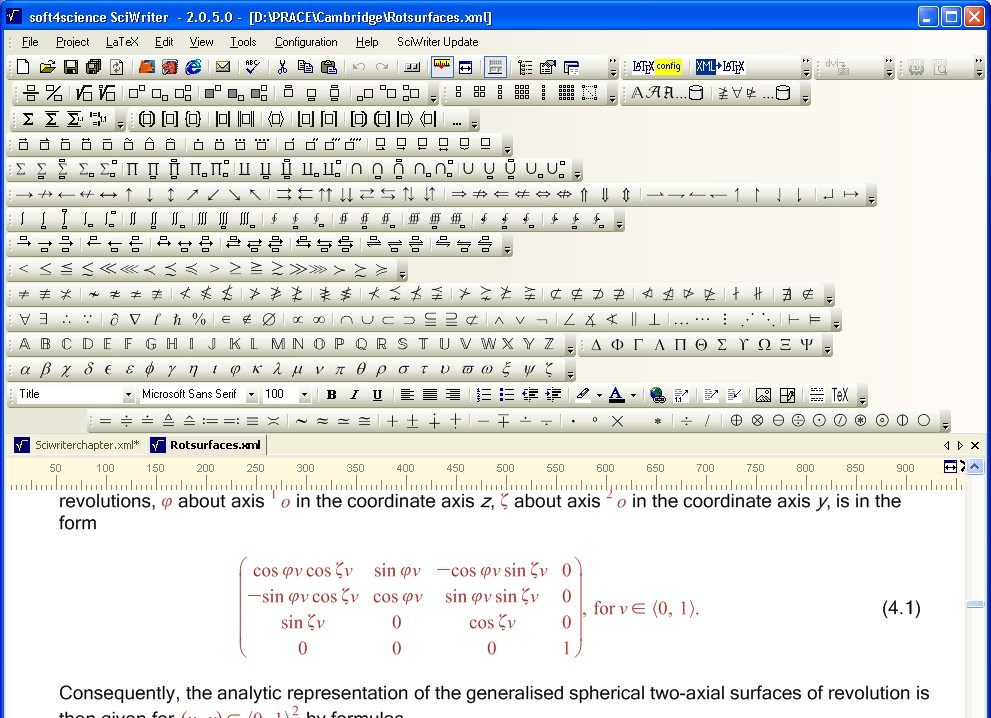Figure 1: Chart of available mathematical symbols

All mathematical symbols and operators can also be entered without using the mouse.

Example 1: Inserting the mathematical "not element" operator: $\notin$ (see Figure 2).

Step 1. Keyboard Input: Alt+O - opens the Insert Operator Dialog

Step 2. Keyboard Input: "notel" - selects the first symbols of the name "not element"

Step 3. Keyboard Input: OK [Return] - Inserts the Symbol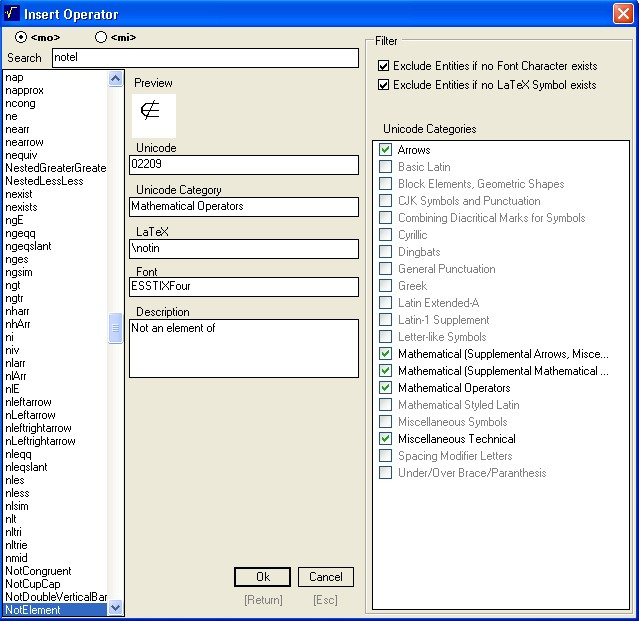Figure 2: Inserting mathematical symbol

To hide or show toolbars open the Customize Toolbar dialogue window and click on the toolbar that you want to hide or show, Figure 3.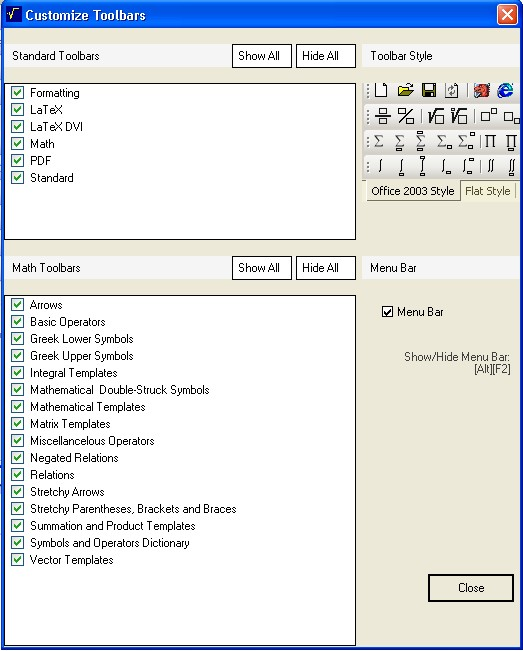Figure 3: Customize Toolbar dialogue window

All document properties of the current active document can be defined in the Document Properties dialogue window (Figure 4) availale from the item View, with the following categories - SciWriter Appearance, Environments, General Properties, General Text, Headings, Latex Export, MetaData, Numbering Schemes, Page Margins, Vertical Margins, Paragraph Margins.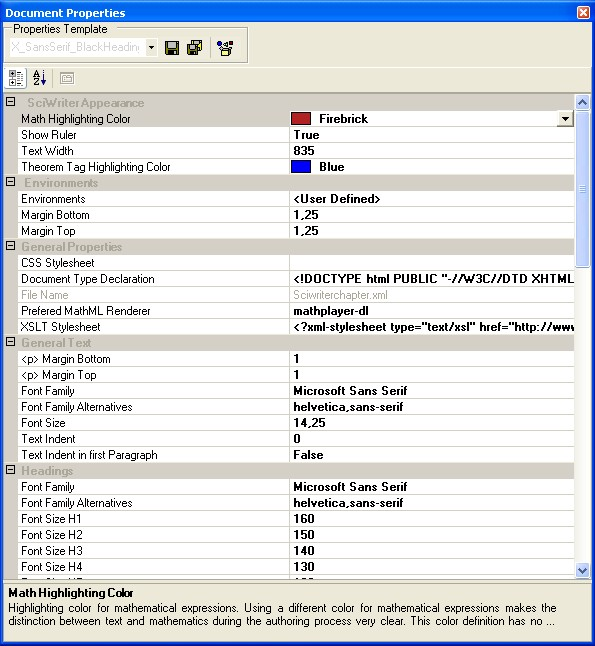Figure 4: Document Properties dialogue window

### SciWriter features

#### Interoperability with Computer Algebra Systems

Most Computer Algebra Systems support import and export of MathML. Here we will have a look at the two mathematical packages - Maple from MapleSoft and Mathematica from Wolfram Research.

##### Interoperability with Mathematica

Copy and Paste from SciWriter to Mathematica

Figure 5 shows how easy it is to copy (shortcut: Ctrl+C) an expression of a function of one variable from SciWriter and paste (shortcut: Ctrl+V) it into Mathematica in order to plot a graph of the function.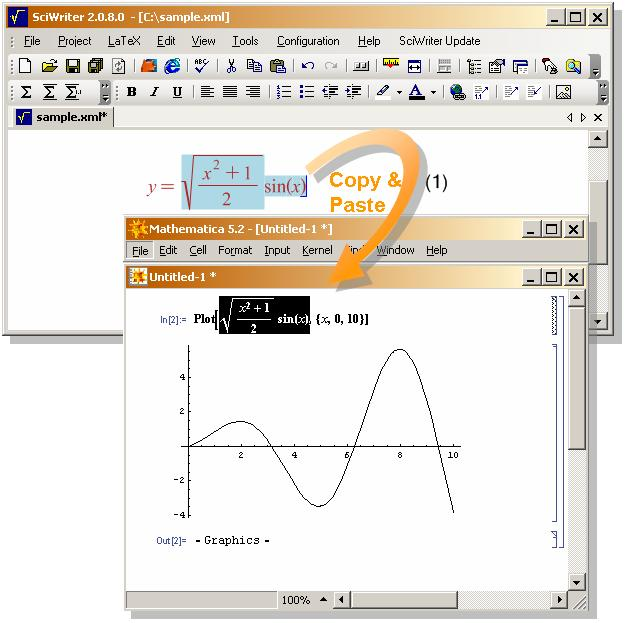Figure 5: Copy and paste

Copy and Paste from Mathematica to SciWriter

To copy a mathematical expression from Mathematica to SciWriter one has to select the expression, right click to open the context menu and choose the sub menu item MathML in the Copy As menu group (see Figure 6).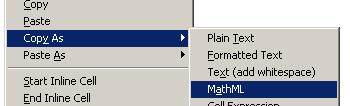Figure 6: Copy form Mathematica
##### Interoperability with Maple

Copy and Paste from SciWriter to Maple

As with Mathematica it is possible to copy and paste mathematical expressions from SciWriter to Maple (see Figure 7) simple by using the standard keyboard commands Ctrl+C and Ctrl+V.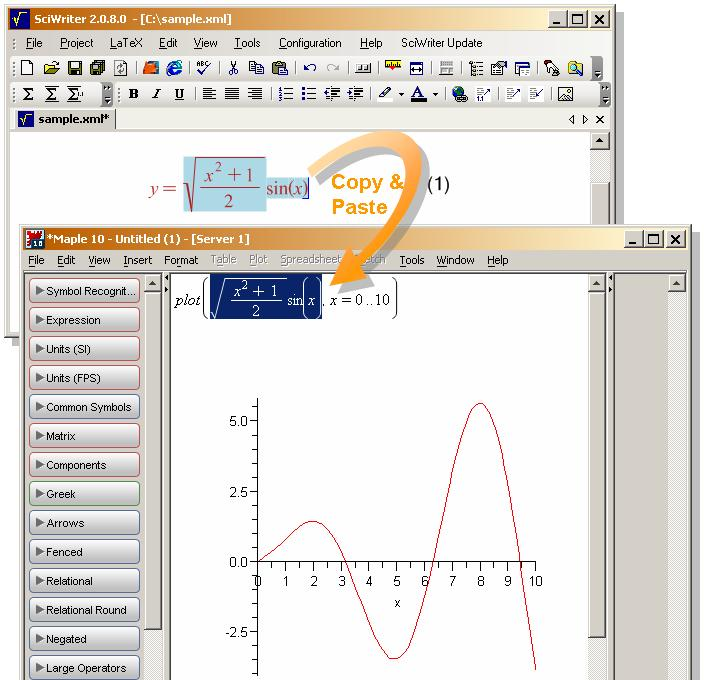Figure 7: Copy and paste

Copy and Paste from Maple to SciWriter

It is also possible to copy and paste expressions (formatted as MathML) from Maple to SciWriter. A selected mathematical expression in Maple can be translated to MathML by exporting as MathML-encoded text. This text is produced in the form of a Maple string which may then be printed or otherwise processed further. Formulas can be converted also with the User Defined Context Menu (Figure 8) to Export MathML Presentation Markup, which is supported by SciWriter.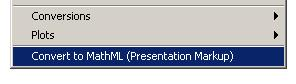Figure 8: Conversion from Maple

Example 2. Exporting formula $a+2\ast b$ from Maple to SciWriter

Step 1. Type command: MathML[ExportPresentation]( a + 2 * b );

Step 2. Copy (shortcut: Ctrl+C) the Maple return - string of MathML encoded formula

"$<\mathrm{math}\phantom{\rule{mediummathspace}{0.2em}}\mathrm{xmlns}=\text{'}\mathrm{http}://\mathrm{www}.w3.\mathrm{org}/1998/\mathrm{Math}/\mathrm{MathML}\text{'}>$$<\mathrm{mrow}><\mathrm{mi}>a<\mathrm{mo}>+$$<\mathrm{mrow}><\mathrm{mn}>2<\mathrm{mo}>&\mathrm{InvisibleTimes};$$<\mathrm{mi}>b$"

Step 3. Paste (shortcut: Ctrl+V) the copied string to SciWriter, where the encoded formula appears in the form

"$a+2b$"

Whenever a mathematical expression is copied to the clipboard, it will be encoded as MathML. This allows the user to ''copy and paste'' mathematical expressions from SciWriter to any other application that can interpret MathML.

#### General Authoring Features

Using SciWriter as an editor for production of mathematical documents one can benefit from the following authoring features:

1. Easy-to-use Graphical Interface similar to the most commonly used text editors.
3. Autonumbered Section Headings with the possibility od using a non-numbered selection possible from the first rolldown menu on the left.
4. Autonumbered Figure, Table and Equation Blocks.
5. Comfortable Management of Cross References to Sections, Tables, Figures, Equations, Bibliographic Items, Theorem Environments and Footnotes through the Insert Cross Reference Dialogue opened by a Cross Reference Toolbar button.
6. Multi-Level Undo and Redo functions
7. Customizable Theorem Environments, e.g. for Proof, Axiom, Definition, etc, can be easily selected from the first rolldown menu on the left as specific paragraph style. There are available the following Theorem Environments - Acknowledgement, Algorithm, Axiom, Case, Claim, Comment, Conclusion, Condition, Conjecture, Colloraly, Criterion, Definition, Example, Exercise, Lemma, Notation, Problem, Proof, Proposition, Remark, Solution, Summary, Theorem. Numbered or non-numbered options can be chosen from the Document Properties dialogue window (Figure 4), category Numbering schemes.
8. Spell Checker with combined US and UK English dictionary is available in the Tools menu.

#### LaTeX Export and Print Publishing Features

Export of edited documents to LaTeX is provided by XSLT Transformation on a button click from the top menu XML ->LateX (see Figure 1). It is possible to create TeX/LaTeX documents without any TeX/LaTeX previous experience, while using MikTeX these documents can be published with a high-quality layout. In addition, for each document a LaTeX preamble can be configured individually. The tex files produced support AMS-LaTeX Math Symbols and Theorem Environments.

Web Publishing Features

SciWriter XML Documents are valid against the XHTML 1.1 plus MathML 2.0 DTD and can be published on the web as they are, with mathematical expressions encoded as MathML. Export of the native document to HTML with mathematical expressions converted to Jpeg images is possible automatically, from item File, Save as. The image files will be stored in a subdirectory. The folder name of this subdirectory will be automaticaly created by the main document name and the postfix "_files". The MathML representation of all mathematical expressions will be stored in the comment block of the Jpeg file. When a document that was stored as XHTML+Math Images will be re-opened and edited, SciWriter will extract the MathML code from the comment block of the Math image files. It is therefore also possible to edit those mathematical expressions that were previously saved as image files.

Documents created with SciWriter are valid against the following W3C Recommendations:

1. XML 1.0
2. XHTML 1.1 + MathML 2.0

#### References

 soft4Science, http://www.soft4science.com/products/SciWriter/

 MathML at the W3C, http://www.w3.org/math